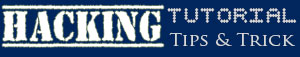# How to Make a Python host checker for LinuxHello. Today we are going to make a Python up/down host checker "How to Make a Python host checker for Linux" that works on Linux only.

You are going to need:

– Python 3.4

– Internet Connection

– Computer with Windows or Linux

You can find some of the Python basics in my first tutorial at http://www.hacking-tutorial.com/hacking-tutorial/code-your-first-simple-sql-injection-checking-vulnerability-with-python/

Why Linux only?

We never recommend using 3rd party libraries, but, without them you cant normally ping on Windows. Like, if you use ping command on Linux, you get 1 if the host is up, and other numbers if it is down. That means, on Linux, when the ping target is not pinged successfully, the ping returns 1 (operation completed successfully), in another case, it gives you the error number. On Windows, this is more complicated. If you ping with ping command, you get operation completed successfully, even if the host is up or down. Howewer, I don’t think any of you actually use Windows for hacking.

Setting up

Before starting, please connect to the internet, and if you would have another computers up, that would be pretty nice. Get your local IPv4 address, think what ports do you want to scan.

## Coding How to Make a Python host checker for Linux

Coding is the easy part. Begin from importing sys and socket, then, write the following code:

`import os # Importing main libs`

`import sys`

`start = "" # Setting up variables`

`range1 = 0`

`range2 = 0`

`for carg in sys.argv: # Checking for arguments`

`    if carg == "-s":`

`        argnum = sys.argv.index(carg)`

`        argnum += 1`

`        start = sys.argv[argnum]`

`    elif carg == "-r1":`

`        argnum = sys.argv.index(carg)`

`        argnum += 1`

`        range1r = sys.argv[argnum]`

`        range1 = int(range1r)`

`    elif carg == "-r2":`

`        argnum = sys.argv.index(carg)`

`        argnum += 1`

`        range2r = sys.argv[argnum]`

`        range2 = int(range2r)`

`print ("[*] Host Scanner launched!") # Informs user about initialize`

`if start == "": # Checks if all the information is provided`

`    print ("[E] No host provided")`

`elif range1 == 0:`

`    print ("[E] No range1 provided")`

`elif range2 == 0:`

`    print ("[E] No range2 provided")`

`else:`

`    if range1 > range2:`

`        count = range1 - range2`

`    elif range1 < range2:`

`        count = range2 - range1`

`    for ccount in range(range1, range2): # Counts the IP range to ping`

`            target = start + "." + str(ccount)`

`            response = os.system("ping " + target + " 2>&1 >/dev/null") # Sets response to ping`

`            if response == 0: # Reads response, checks if it is 0`

`                err = 0 # sets err to 0`

`            else:`

`                err = 1 # sets err to 1`

`            if err == 0: # when err is equal to 0`

`                print ("[+] " + target + " is up!") # Informs user about hosts that are up`

Code should look like this (comments are cut, do not worry):So, that is pretty easy. The end perimeters in th ping command supresses the commands output. So, save the file, run it from terminal and test this out!